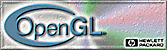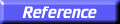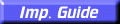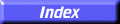# gluCylinder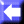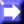gluCylinder: draw a cylinder.

##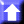C Specification

```void gluCylinder(
GLdouble	 base,
GLdouble	 top,
GLdouble	 height,
GLint	 slices,
GLint	 stacks)
```

##Parameters

base
Specifies the radius of the cylinder at z = 0.
top
Specifies the radius of the cylinder at z = height.
height
Specifies the height of the cylinder.
slices
Specifies the number of subdivisions around the Z axis.
stacks
Specifies the number of subdivisions along the Z axis.

##Description

gluCylinder draws a cylinder oriented along the Z axis. The base of the cylinder is placed at Z = 0, and the top at Z = height. Like a sphere, a cylinder is subdivided around the Z axis into slices, and along the Z axis into stacks.

Note that if top is set to 0.0, this routine generates a cone.

If the orientation is set to GLU_OUTSIDE (with gluQuadricOrientation), then any generated normals point away from the Z axis. Otherwise, they point toward the Z axis.

If texturing is turned on (with gluQuadricTexture), then texture coordinates are generated so that t ranges linearly from 0.0 at Z = 0 to 1.0 at Z = height, and s ranges from 0.0 at the +Y axis, to 0.25 at the +X axis, to 0.5 at the
Y axis, to 0.75 at theX axis, and back to 1.0 at the +Y axis.

gluDisk,